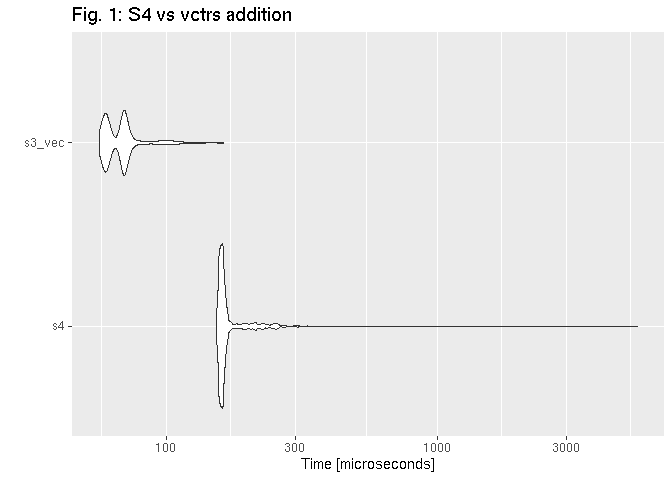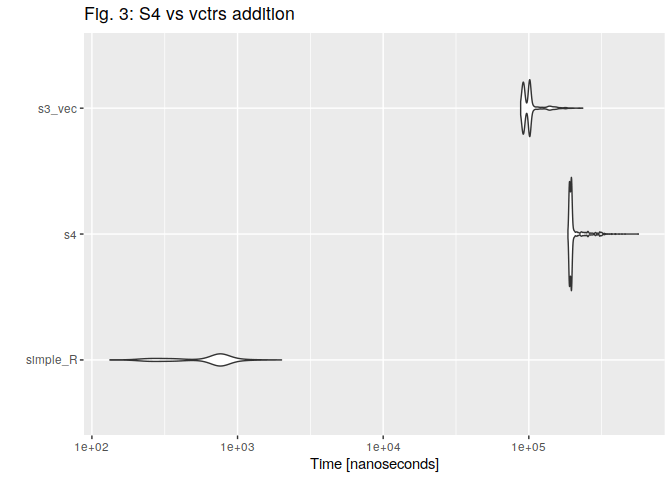Want to share your content on R-bloggers? click here if you have a blog, or here if you don't.## Remake

About two weeks ago, I published on my blog a comparision between two possible implementation of double-dispatch: S4-based and vctrs-based. It turned out, that in my trials vctrs performed better. However, two days later I’ve got a message from Lionel Henry via comment to my commit on GitHub. He suggested, that S4 got faster in the newest versions of R.I decided to remake this experiment and check out, if my findings are still true.

## Why do we may need double dispatch?

In most cases, when writing R scripts or even creating R packages, it is enough to use standard functions or S3 methods. However, there is one important field that forces us to consider double dispatch question: arithmetic operators.

Suppose we’d like to create a class, which fits the problem we’re currently working on. Let’s name such class beer.

```beer <- function(type){
structure(list(type  = type),class = "beer")
}

opener <- function(){
structure(list(), class = "opener")
}

pilsner <- beer("pilnser")
my_opener <- opener()
```

Then, we create an operator which defines some non-standard behaviour.

• if we add an opener to the beer, we get an opened_beer.
• adding a numeric x, we get a case of beers (which even contain a negative number of bees, i.e. our debt…)
• if second argument is different than a or opener or numeric, we get… untouched beer

Let’s demonstrate, how does it work:

````+.beer` <- function(a, b){
if (inherits(b, "opener")) {
return(structure(list(
name  = paste("opened", a\$name)
), class = "opened_beer"))
} else if (inherits(b, "numeric")) {
print("It's magic! You've got a case of beers!")
return(structure(list(
n_beers = 1 + b
), class = "case_of_beers"))
} else {
return(a)
}
}

pilsner + my_opener

## \$name
##  "opened "
##
## attr(,"class")
##  "opened_beer"

pilsner + -0.1

##  "It's magic! You've got a case of beers!"

## \$n_beers
##  0.9
##
## attr(,"class")
##  "case_of_beers"
```

Don’t you think, that such operations should be commutative?

```my_opener + pilsner

## list()
## attr(,"class")
##  "opener"
```

What did happen here? This is an example of the way the R interpreter handles arithmetic operator. It was described with details on Hiroaki Yutani’s blog. Briefly speaking, in this particular case R engine matched method to the second argument (not to the first one), because there is no `+.opener` S3 method. What about such trick:

````+.opener` <- function(a, b) b + a
```

After that, the result is different:

```my_opener + pilsner

## Warning: Incompatible methods ("+.opener", "+.beer") for "+"

## Error in my_opener + pilsner: non-numeric argument to binary operator
```

We crashed our function call. When both objects have the `+` method defined and these methods are not the same, R is trying to resolve the conflict by applying an internal `+`. It obviously cannot work. This case could be easily solved using more ‘ifs’ in the `+.beer` beer function body. But let’s face a different situation.

```-0.1 + pilsner

##  -0.1
```

What a mess! Simple S3 methods are definitely not the best solution when we need the double dispatch.

## S4 class: a classic approach

To civilize such code, we can use classic R approach, S4 methods. We’ll start from S4 classes declaration.

```.S4_beer          <- setClass("S4_beer", representation(type = "character"))
.S4_opened_beer   <- setClass("S4_opened_beer", representation(type = "character"))
.S4_opener        <- setClass("S4_opener", representation(ID = "numeric"))
.S4_case_of_beers <- setClass("S4_case_of_beers", representation(n_beers = "numeric"))
```

Then, we can two otptions, how to handle `+` operators. I didn’t mention about it in the previous example, but both S3 and S4 operators are grouped as so-called group generic functions (learn more: S3, S4).

We can set a S4 method for a single operator and that looks as follows:

```setMethod("+", c(e1 = "S4_beer", e2 = "S4_opener"),
function(e1, e2){
if (inherits(e2, "S4_opener")) {
return(.S4_opened_beer(type  = paste("opened", e1@type)))
} else if (inherits(e2, "numeric")) {
print("It's magic! You've got a case of beers!")
return(.S4_case_of_beers(n_beers = 1 + e2))
} else {
return(e1)
}
})

setMethod("+", c(e1 = "S4_opener", e2 = "S4_beer"),
function(e1, e2) e2 + e1)
```

Alternatively, we can define a method for `Arith` geneneric and check, what method is exactly called at the moment. I decided to use the second approach, because it’s more similar to the way the double dispatch is implemented in the vctrs library.

```.S4_fun <- function(e1, e2){
if (inherits(e2, "S4_opener")) {
return(.S4_opened_beer(type  = paste("opened", e1@type)))
} else if (inherits(e2, "numeric")) {
print("It's magic! You've got a case of beers!")
return(.S4_case_of_beers(n_beers = 1 + e2))
} else {
return(e1)
}
}

setMethod("Arith", c(e1 = "S4_beer", e2 = "S4_opener"),
function(e1, e2)
{
op = .Generic[]
switch(op,
`+`  = .S4_fun(e1, e2),
stop("undefined operation")
)
})

setMethod("Arith", c(e1="S4_opener", e2="S4_beer"),
function(e1, e2)
{
op = .Generic[]
switch(op,
`+`  = e2 + e1,
stop("undefined operation")
)
})
```

Let’s create our class instances and do a piece of math.

```S4_pilsner <- .S4_beer(type = "Pilsner")
S4_opener <- .S4_opener(ID = 1)

S4_pilsner + S4_opener

## An object of class "S4_opened_beer"
## Slot "type":
##  "opened Pilsner"

S4_opener + S4_pilsner

## An object of class "S4_opened_beer"
## Slot "type":
##  "opened Pilsner"
```

Declared methods are clear, and, the most important: they work correctly.

## vctrs library: a tidyverse approach

vctrs is an interesting library, thought as a remedy for a couple of R disadvantages. It delivers, among others, a custom double-dispatch system based on well-known S3 mechanism.

At the first step we declare class ‘constructors’.

```library(vctrs)

.vec_beer <- function(type){
new_vctr(.data = list(type  = type), class = "vec_beer")
}

.vec_opened_beer <- function(type){
new_vctr(.data = list(type  = type), class = "vec_opened_beer")
}

.vec_case_of_beers <- function(n_beers){
new_vctr(.data = list(n_beers  = n_beers), class = "vec_case_of_beers")
}

.vec_opener <- function(){
new_vctr(.data = list(), class = "vec_opener")
}
```

Then, we create class instances.

```vec_pilsner   <- .vec_beer("pilnser")
vec_opener <- .vec_opener()
print(class(vec_pilsner))

##  "vec_beer"   "vctrs_vctr" "list"

print(class(vec_opener))

##  "vec_opener" "vctrs_vctr" "list"
```

At the end, we write a double-dispatched methods in vctrs style. As you can see,

```.fun <- function(a, b){
if (inherits(b, "vec_opener")) {
return(.vec_opened_beer(type  = paste("opened", a\$type)))
} else if (inherits(b, "numeric")) {
print("It's magic! You've got a case of beers!")
return(.vec_case_of_beers(n_beers = 1 + b))
} else {
return(a)
}
}

vec_arith.vec_beer <- function(op, x, y, ...) {
UseMethod("vec_arith.vec_beer", y)
}

vec_arith.vec_opener <- function(op, x, y, ...) {
UseMethod("vec_arith.vec_opener", y)
}

vec_arith.vec_beer.vec_opener <- function(op, x, y, ...){
switch(op,
`+` = .fun(x, y),
stop_incompatible_op(op, x, y)
)
}

vec_arith.vec_opener.vec_beer <- function(op, x, y, ...){
y + x
}

vec_pilsner + vec_opener

## <vec_opened_beer>
##           type
## opened pilnser

vec_opener + vec_pilsner

## <vec_opened_beer>
##           type
## opened pilnser
```

It works properly, too.

## Benchmark

I’ve created all the classes and methods above not only to demonstate, how to implement double dispatch in R. My main goal is to benchmark both approaches and check, which one has smaller overhead. The hardware I used for the test looks as follows:

```## \$vendor_id
##  "GenuineIntel"
##
## \$model_name
##  "Intel(R) Core(TM) i3 CPU       M 350  @ 2.27GHz"
##
## \$no_of_cores
##  4

## 8.19 GB

sessionInfo()

## R version 4.0.2 (2020-06-22)
## Platform: x86_64-pc-linux-gnu (64-bit)
## Running under: Ubuntu 18.04.2 LTS
##
## Matrix products: default
## BLAS/LAPACK: /opt/intel/compilers_and_libraries_2018.2.199/linux/mkl/lib/intel64_lin/libmkl_rt.so
##
## locale:
##   LC_CTYPE=pl_PL.UTF-8       LC_NUMERIC=C
##   LC_TIME=pl_PL.UTF-8        LC_COLLATE=pl_PL.UTF-8
##   LC_MONETARY=pl_PL.UTF-8    LC_MESSAGES=en_US.utf8
##   LC_PAPER=pl_PL.UTF-8       LC_NAME=C
##  LC_MEASUREMENT=pl_PL.UTF-8 LC_IDENTIFICATION=C
##
## attached base packages:
##  stats     graphics  grDevices utils     datasets  methods   base
##
## other attached packages:
##  vctrs_0.3.4
##
## loaded via a namespace (and not attached):
##   benchmarkmeData_1.0.4 knitr_1.30            magrittr_1.5
##   tidyselect_1.1.0      doParallel_1.0.15     lattice_0.20-41
##   R6_2.4.1              rlang_0.4.7           foreach_1.5.0
##  httr_1.4.2            stringr_1.4.0         dplyr_1.0.2
##  tools_4.0.2           parallel_4.0.2        grid_4.0.2
##  xfun_0.18             ellipsis_0.3.1        htmltools_0.5.0
##  iterators_1.0.12      yaml_2.2.1            digest_0.6.25
##  tibble_3.0.3          benchmarkme_1.0.4     lifecycle_0.2.0
##  crayon_1.3.4          Matrix_1.2-18         purrr_0.3.4
##  codetools_0.2-16      glue_1.4.2            evaluate_0.14
##  rmarkdown_2.4         stringi_1.5.3         pillar_1.4.6
##  compiler_4.0.2        generics_0.0.2        pkgconfig_2.0.3
```

It’s my good old notebook, which is not a beast.

```library(microbenchmark)
library(ggplot2)
```

### Beer + opener

```bm1 <- microbenchmark(
s4 = S4_pilsner + S4_opener,
s3_vec = vec_pilsner + vec_opener,
times = 1000
)
```

#### R 4.0.2

```## Unit: microseconds
##    expr     min       lq      mean  median       uq      max neval
##      s4 177.111 182.9710 197.09576 186.997 190.7615 1937.005  1000
##  s3_vec  68.568  74.3705  83.27131  82.710  84.4995  306.320  1000
```#### R 3.6.1### Opener + beer

```bm2 <- microbenchmark(
s4 = S4_opener + S4_pilsner,
s3_vec = vec_opener + vec_pilsner,
times = 1000
)
```

#### R 4.0.2

```## Unit: microseconds
##    expr     min       lq     mean   median       uq      max neval
##      s4 186.736 191.6060 216.6038 196.0305 200.1195 8109.925  1000
##  s3_vec  87.808  92.0705 110.3604 100.2730 102.7115 6588.121  1000
```#### R 3.6.1### Bonus: opener + beer vs addtion of numerics

```bm3 <- microbenchmark(
simple_R = 1 + 2,
s4 = S4_opener + S4_pilsner,
s3_vec = vec_opener + vec_pilsner,
times = 1000
)
```

#### R 4.0.2

```## Unit: nanoseconds
##      expr    min       lq       mean   median       uq    max neval
##  simple_R    133    374.0    643.296    722.0    795.0   2004  1000
##        s4 185652 190964.5 206643.130 195424.0 198756.5 564443  1000
##    s3_vec  87889  92022.0 103274.276 100360.5 102306.0 234482  1000
```#### R 3.6.1## Conclusions

Results are roughly the same as outcomes of my previous experiments run on R 3.6.1. We can state thar regarding these particular machine and examples, vctrs-based performs better than S4 methods.

## Further sources

If you are interesting, how to implement double-dispatched operators in S4, I encourage you to get familiar with code of the following R libraries:

If you are looking for some examples of vctrs, I recommend you to learn the source code of: